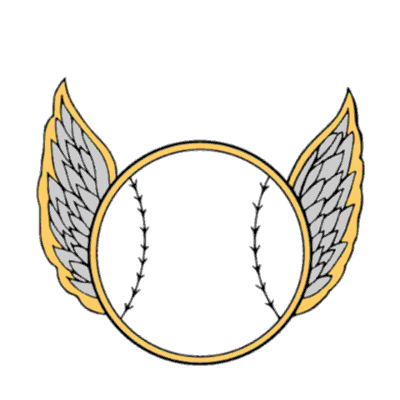# Field calculate sequential letters

3667
8
12-13-2017 02:20 PMby
New Contributor

Hello,

I am trying to figure out how I can use the field calculator with python to calculate sequential letters. For example, I would like "A,B,C,D....Z, AA,AB, AC...".  I did find a code that works just fine in a regular python environment but I am having a hard time incorporating it on the field calculator.

I tried using this as the code block:

"""

from string import ascii_lowercase
import itertools

def iter_all_strings():
size = 1
while True:
for s in itertools.product(ascii_lowercase, repeat=size):
yield "".join(s)
size +=1

"""

and  "iter_all_strings()" as the expression in the field calculator but it just gives me null values.

Any help would be much appreciated, thank you.

1 Solution

Accepted SolutionsbyMVP Regular Contributor

And using your code, this would go in the code block:

``````from string import ascii_lowercase
import itertools

def iter_all_strings():
size = 1
while True:
for s in itertools.product(ascii_lowercase, repeat=size):
yield "".join(s)
size +=1

s = iter_all_strings()
‍‍‍‍‍‍‍‍‍‍‍‍‍‍‍‍‍‍‍‍‍‍‍``````

The expression would be:

``s.next()‍‍``

The generator needs to be set up in the code block.

8 RepliesbyMVP Esteemed Contributor

how big is the file? aka does it cut off at ZZ?  If it is short, it might just be easier to generate the list, then slice it based on a unique ID field (perhaps OBJECTID -1 )New Contributor III

This is fairly straightforward.  Sorry, I don't have the time to write the code for you but if you check the end of this video I did, you can see how I used Python for creating the letters: https://www.youtube.com/watch?v=QA0iFlP0V3Y - I needed A1 to AA1 to AAA1, etc. If you're really stuck and still can't work it out, let me know and I can create a working example for you.byMVP Regular Contributor

Using a modified number base function, try this in the code block:

``````i = -1

def letterString():
global i
i += 1

def toStr(n,base):
convertString = "ABCDEFGHIJKLMNOPQRSTUVWXYZ"
if n < base:
return convertString[n]
else:

and this for the expression

``letterString()‍‍``

Basically, you will need to set up a global variable in your code block and increment it each time your function is called.byMVP Regular Contributor

And using your code, this would go in the code block:

``````from string import ascii_lowercase
import itertools

def iter_all_strings():
size = 1
while True:
for s in itertools.product(ascii_lowercase, repeat=size):
yield "".join(s)
size +=1

s = iter_all_strings()
‍‍‍‍‍‍‍‍‍‍‍‍‍‍‍‍‍‍‍‍‍‍‍``````

The expression would be:

``s.next()‍‍``

The generator needs to be set up in the code block.by
New Contributor

Randy,

Thank you very much, problem solved!byMVP Esteemed Contributor

Expression:  next(values)

Expression Type:  PYTHON_9.3

Code Block:

``````from itertools import count, product
from string import ascii_lowercase

cnt = count(1)
values = ("".join(v)
for c in cnt
for v in product(ascii_lowercase, repeat=c))‍‍‍‍‍‍‍``````by
Regular Contributor II
``````from string import ascii_uppercase
import itertools

def iter_all_strings():
size = 1
while True:
for s in itertools.product(ascii_uppercase, repeat=size):
yield "".join(s)
size +=1

for s in itertools.islice(iter_all_strings(), 54):
print s‍‍‍‍‍‍‍‍‍‍‍‍``````

Just save your stopping point in line 11 (number of iterations you want  54... I believe is "BB"byMVP Regular Contributor

A clean, efficient solution.  Thanks for sharing bixb0012‌.